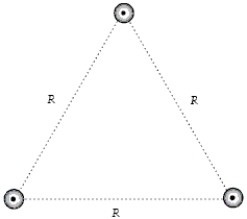# [Solved] The Figure Shows a Cross Section of Three Parallel Wires

Question 32
Multiple Choice

## The figure shows a cross section of three parallel wires each carrying a current of 5.0 A out of the paper. If the distance R = 6.0 mm, what is the magnitude of the magnetic force on a 2.0-m length of any one of the wires?A)2.5 mN
B)3.3 mN
C)2.2 mN
D)2.9 mN
E)1.7 mN

10+ million students use Quizplus to study and prepare for their homework, quizzes and exams through 20m+ questions in 300k quizzes.

### Physics & Astronomy

Explore our library and get Introduction to Physical Science Homework Help with various study sets and a huge amount of quizzes and questions

120

Study sets

3.1K

Quizzes

233.2K

Questions

Upload material to get free accessInvite a friend and get free accessSubscribe and get an instant access